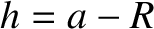Next: Major planetary satellites in Up: Lunar motion Previous: Lunar motion

# Introduction

The orbital motion of the planets around the Sun is fairly accurately described by Kepler's laws. (See Chapter 4.) Similarly, to a first approximation, the orbital motion of the Moon around the Earth can also be accounted for via these laws. However, unlike the planetary orbits, the deviations of the lunar orbit from a Keplerian ellipse are sufficiently large that they are easily apparent to the naked eye. Indeed, the largest of these deviations, which is generally known as evection, was discovered in ancient times by the Alexandrian astronomer Claudius Ptolemy (90 CE-168 CE) (Pannekoek 2011). Moreover, the next largest deviation, which is called variation, was first observed by Tycho Brahe (1546-1601) without the aid of a telescope (Godfray 1853). Another non-Keplerian feature of the lunar orbit, which is sufficient obvious that it was known to the ancient Greeks, is the fact that the lunar perigee (i.e., the point of closest approach to the Earth) precesses (i.e., orbits about the Earth in the same direction as the Moon) at such a rate that, on average, it completes a full circuit everyyears.11.1 The ancient Greeks also noticed that the lunar ascending node (i.e., the point at which the Moon passes through the plane of the Earth's orbit around the Sun from south to north) regresses (i.e., orbits about the Earth in the opposite direction to the Moon) at such a rate that (on average) it completes a full circuit every 18.6 years (Pannekoek 2011). Of course, according to standard two-body orbit theory, the lunar perigee and ascending node should both be stationary. (See Chapter 4.)

Newton demonstrated, in Book III of his Principia, that the deviations of the lunar orbit from a Keplerian ellipse are due to the residual gravitational influence of the Sun, which is sufficiently large that it is not completely negligible compared with the mutual gravitational attraction of the Earth and the Moon. However, Newton was not able to give a full account of these deviations (in the Principia), because of the complexity of the equations of motion that arise in a system of three mutually gravitating bodies. (See Chapter 9.) In fact, Alexis Clairaut (1713-1765) is generally credited with the first reasonably accurate and complete theoretical explanation of the Moon's orbit to be published. His method of calculation makes use of an expansion of the lunar equations of motion in terms of various small parameters. Clairaut, however, initially experienced difficulty in accounting for the precession of the lunar perigee. Indeed, his first calculation overestimated the period of this precession by a factor of about two, leading him to question Newton's inverse-square law of gravitation. Later, he realized that he could account for the precession in terms of standard Newtonian dynamics by continuing his expansion in small parameters to higher order. (See Section 11.17.) After Clairaut, the theory of lunar motion was further elaborated in major works by D'Alembert (1717-1783), Euler (1707-1783), Laplace (1749-1827), Damoiseau (1768-1846), Plana (1781-1864), Poisson (1781-1840), Hansen (1795-1874), De Pontécoulant (1795-1874), J. Herschel (1792-1871), Airy (1801-1892), Delaunay (1816-1872), G.W. Hill (1836-1914), and E.W. Brown (1836-1938) (Brown 1896). The fact that so many celebrated mathematicians and astronomers devoted so much time and effort to lunar theory is a tribute to its inherent difficulty, as well as its great theoretical and practical interest. Indeed, for a period of about one hundred years (between 1767 and about 1850) the so-called method of lunar distance was the principal means used by mariners to determine terrestrial longitude at sea (Cotter 1968). This method depends crucially on a precise knowledge of the position of the Moon in the sky as a function of time. Consequently, astronomers and mathematicians during the period in question were spurred to make ever more accurate observations of the Moon's orbit, and to develop lunar theory to greater and greater precision. An important outcome of these activities were various tables of lunar motion (e.g., those of Mayer, Damoiseau, Plana, Hansen, and Brown), the majority of which were published at public expense.

This chapter contains an introduction to lunar theory in which, amongst other things, approximate expressions for evection, variation, the precession of the perigee, and the regression of the ascending node, are derived from the laws of Newtonian mechanics. Further information on lunar theory can be obtained from Godfray (1853), Brown (1896), Adams (1900), and Cook (1988).Next: Major planetary satellites in Up: Lunar motion Previous: Lunar motion
Richard Fitzpatrick 2016-03-31